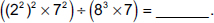# User ForumSubject :IMO    Class : Class 7

Fill in the blanks.
(i) A number is said to be in the _____ form, if it is expressed as the product of a number between 1 and 10 and the integral power of 10.
(ii) 20 × 30 + 10 × 3 = _____.
(iii)(i) (ii) (iii) A Standard 4 7 × 2–5 B Exponential 4 7 × 2–4 C Exponential 2 7 × 25 D Standard 2 7 × 24

How?.....??..

Class : Class 8

## Ans 2:

Class : Class 7
Hey there! The correct option is (A).(i).We know that for any real number K from 1 to 10; when it is multiplied by an integral exponent of 10, is known as the standard form of that number. That is of the form K*10^n for any integer n. So the answer of the first one is standard form.(ii).(2^0)*(3^0) (1^0)*3 = 1*1 1*3 = 1 3 = 4.(iii).((2^2)^2*7^2)/(8^3*7) = (2^4*7^2)/(2^9*7) = 2^(4-9)*7^(2-1) = 7*2^(-5).

Class : Class 8
Howw